Browse by Topic
Related Topics

# Is There Any Point to the 12 Times Table?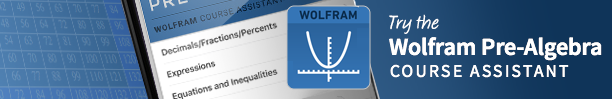My government (I’m in the UK) recently said that children here should learn up to their 12 times table by the age of 9. Now, I always believed that the reason why I learned my 12 times table was because of the money system that the UK used to have—12 pennies in a shilling. Since that madness ended with decimalization the year after I was born, by the late 1970s when I had to learn my 12 times table, it already seemed to be an anachronistic waste of time.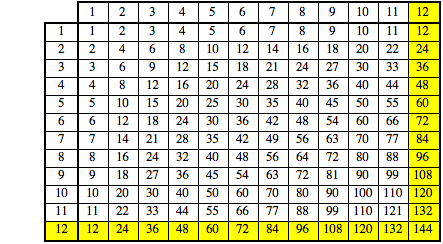To find it being given new emphasis nearly 40 years later struck me as so odd that I thought I should investigate it a little more mathematically. Here is what I concluded.

Let’s start with a basic question: exactly why do we use times tables at all? (This is the kind of question my work on computerbasedmath.org has me asking a lot!)

I am going to claim that there are three basic reasons:

1) To directly know the answer to common multiplication questions.
2) To enable multiplication algorithms.
3) To enable approximate multiplication.

Let’s look at those in turn.

1) This reason is important. There are lots of small multiplication problems in day-to-day life, and there is no doubt that knowing the answer to these is useful. But knowing ANY answer to ANY question is useful. What’s so special about multiplying 1 to 12? Why stop at the 12 times table—why not learn 13, 14, 15, 16, and 17 times tables? Why not learn your 39 times table? As the table number goes up, the amount to learn increases as a square of the number while the commonality of encountering a problem that uses that table goes down. “Knowing” the answer to all possible questions is a big task and not worth the effort. This, after all, is why math was invented, so that we don’t have to know the answers to all possible calculations, but instead have a way to work them out when needed. We must draw a line somewhere and then move to a more algorithmic approach. The question is where.

2) There are many fancy computation algorithms, but most of us learn “multiplying in columns,” which involves operating on one digit at a time while managing number place and carrying overflows on to the next column. I still use it sometimes myself. By definition it needs the 0–9 times tables (and implicitly understanding the 10 times table), since it only takes one digit at a time, but any single digit could come up. Knowledge of 11 and 12 times tables is completely irrelevant. If this was the only consideration, we have a clear argument for where to draw our line—at the 10 times table. You can’t manage on less, and more is of no use.

3) But there is another useful algorithm, which is approximating numbers to a few significant digits. This might make a case for drawing the line higher.

Take as an example 7,203 x 6,892. If I want to know that exactly, then I reach for Mathematica (or if I absolutely have to, I reach for pencil and paper to apply multiplication in columns). But often I just need a rough answer, so I mentally convert this to 7,000 x 7,000 = 7 x 7 x 1,000 x 1,000 = 49,000,000. More formally I am converting the numbers the nearest approximation of the form k x 10n where k ∈ {the set of numbers for which I know times tables}. Then I use the times tables on the remaining significant digits and implicitly use the 10 times table to get the magnitude right. In this case the real answer is:

7203×6892

49643076

Giving me an error of 1.2%—good enough for lots of applications. Now if I knew my 72 times table, I could have made this 7,200 x 6,900 = 49,680,000. Only a 0.07% error.

So, now our “where do I draw the line” question becomes “how much better is a typical approximate calculation if I know up to the 12 times table compared to only knowing my 10 times table?” Let’s investigate. First I need to automate the process of approximating using a given lead number.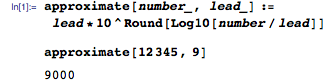And extend that to finding the best approximation, if we have a choice of lead numbers.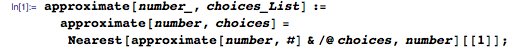For example, if I know only up to my 4 times table, then the best approximation for 18,345 is 20,000.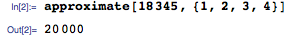Now our approximate product is just the product of the best approximations of each number.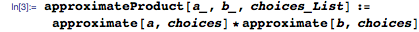And the relative error can be found from the difference compared to the accurate answer.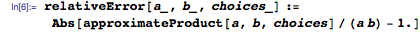For example, working out 549 x 999 when you only know up to your 10 times table will give you a little over an 8% error.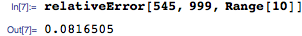Now, let’s take “typical calculation” to mean a calculation involving uniformly distributed numbers between 1 and 1 million and take the “typical” error to be the average of 100,000 such calculations.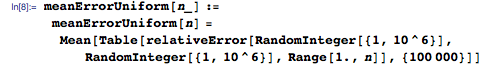The typical error if you know up to your 10 times table is 9.4%.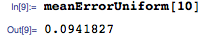But if you know up to your 12 times table, it is only 8.2%.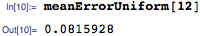Here is the error as a function of how many of your times tables you have learned.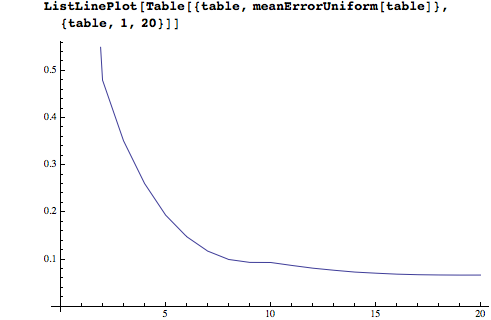Interestingly, most of the improvement happens by the time you know your 7 times table. The odd bump at 10 is because the ability to approximate relies implicitly on knowing your 10 times table already (to be able to handle the trailing zeros).

We can work out how much relative improvement there is in the typical error for each extra table learned.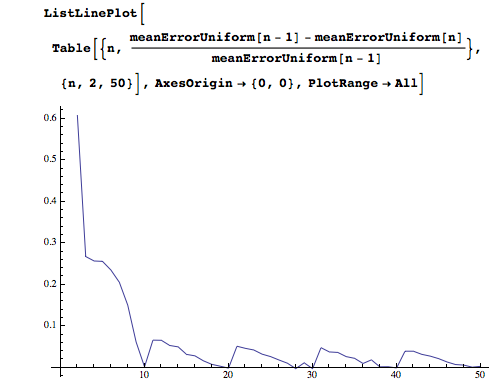So the relative benefit gradually drops, in a cyclic way.

But the improvement in error from 9% to 8% comes at a price. Knowing up to your 10 times table requires recollection of 100 facts (OK, 55, if you assume symmetry). But knowing up to your 12 times table is 144 facts. Improving the error from 9.3% of the result to 8.1% is a relative improvement of 12% in the size of the error. But to achieve that you need to memorize 40% more information. That seems like a losing proposition.

Let’s look at the relative improvement in outcome, per extra fact memorized.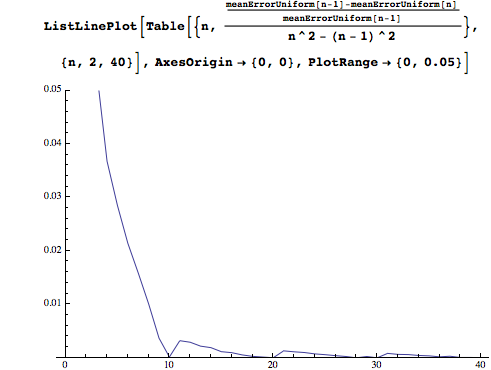The “return on effort” drops very rapidly toward the 10 times table and then barely improves. It seems like a fairly compelling case for stopping our rote learning at 10. Indeed, if times tables were only for estimating, we would get the best return per effort by just looking at the orders of magnitude and using only the 1 and 10 times tables!

Of course numbers are not uniformly distributed. If you are in egg production, 6s and 12s probably come up a lot, just as they will if you happen to be a dealer in pre-decimal British coins! Context issues like these are hard to quantify, but one issue that is general is Benford’s law, which occurs in many real-world datasets. It says that if you look at real-life datasets that cover several orders of magnitude (e.g. populations of communities, or people’s debts, or file sizes on your computer), then the numbers are more likely to start with a 1 than with a 2, and more likely to start with a 2 than a 3, and so on. I don’t know if anyone has studied the distribution of second digits, so I will assume that is uniform. So here is a function that generates “real-world” numbers.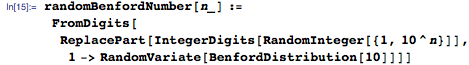We can now repeat our analysis on these more realistic numbers.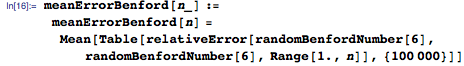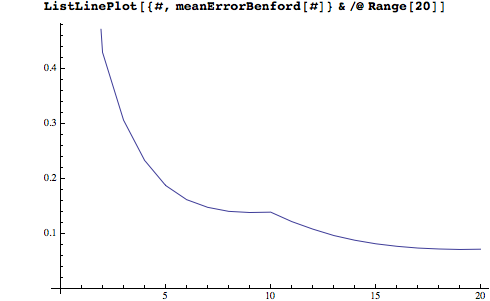Using these less uniform numbers gives poorer performance (making you more likely to need accurate computation rather than approximation). Improvement can still be achieved by knowing more tables, and this could be taken as an argument for learning beyond 12, but not when you take into account the return per extra fact learned, which makes an even stronger argument for stopping at 10.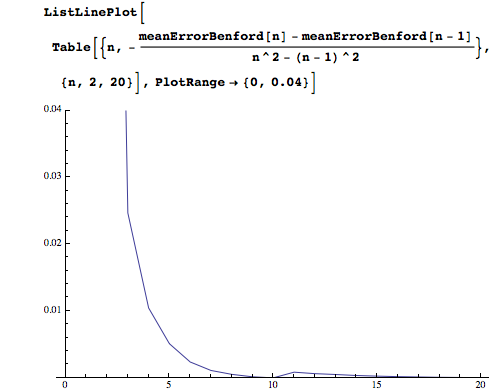If you really are intent on some extra rote learning, there are better ways to spend your effort than learning 11 and 12 times tables. Learning all permutations of 1 to 10 together with 15 and 25 gives a better average result than 1 to 12 (since they more evenly approximate the numbers with a lead digit of 1 or 2).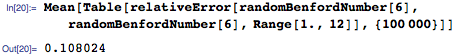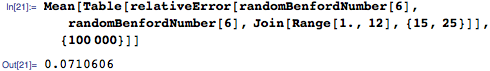Or, as Chris Carlson suggested to me, learn the near reciprocals of 100 (2 x 50 = 100, 3 x 33 = 99, 4 x 25 = 100, 5 x 20 = 100, 6 x 17 = 102, etc.), as they come up a lot. I would expect that learning squares and powers of 2 is also probably more useful than 11 and 12 times tables.

With no prospect of the pre-decimal money system returning, I can only conclude that the logic behind this new priority is simply, “If learning tables up to 10 is good, then learning them up to 12 is better.” And when you want to raise standards in math, then who could argue with that? Unless you actually apply some math to the question!